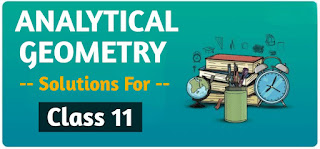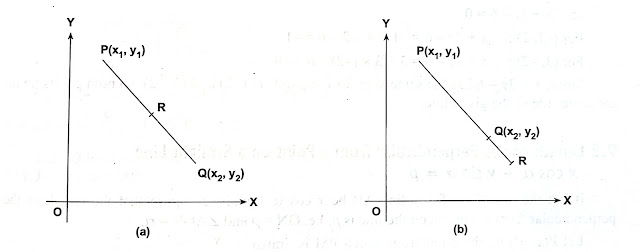# Analytical Geometry Class 11 Mathematics Solutions | Exercise - 9.2## Chapter - 9  Analytical Geometry

We all have to study Analytical Geometry chapter in class 11 mathematics. Though we study about this chapter, many of us don't know what exactly 'Analytical Geometry means. So let me tell you about this chapter a little bit.

Basically Analytical Geometry is a branch of algebra which deals with the modelling of some geometrical objects such as curves, lines,plots and so on. In this chapter we will study about various subtopics falling under it. So let's have a quick look at them too.

### Review of Equations of Straight Lines

The equation of the line parallel to x-axis is x = a When a = 0, x = 0 will be the equation of y-axis. In the same way, the equation of the line parallel to y-axis is y = b. If b = 0 , then y = 0 will be the equation of the x-axis.

Three standard forms of equation of a line

Three standard forms of equation of a line are given below:

• y = mx + c, is known as the slope-intercept form where m and c are the slope and the y- intercept respectively.
• x/a + y/b = 1, is known as the double-intercept form where a and b are the x-intercept and y- b intercept respectively.
• xcosα + ysinα = p is known as the normal form or perpendicular form where p is the perpendicular from origin to the line and a, the angle made by the perpendicular with x- axis.

Equations of lines in spacial cases:

The following are the two special forms of a line:

• Point slope form: y - y₁ = m(x - x₁) where m is the slope of the line through (x₁, y₁).
• Two points form: y - y₁ = {(y₂ - y₁) / (x₂ - x₁)} (x - x₁) is the line through two given points (x₁, y₁) and (x₂,y₂)

### Linear Equation

The general equation of first degree in x and y is Ax + By + C = 0 where A, B and C constants and A, B are not simultaneously zero. This equation is called the linear equation. This equation always represents a straight line

### Important Points of Concurrencies

The following are the four important definitions of point of concurrencies of three straight lines.

i) Orthocentre: The perpendiculars drawn from the vertices on their opposite sides meet at a point. This point is known as 'Orthocentre'.

ii) Circumcentre: The perpendicular bisectors of the sides of a triangle meet at a point. This point is known as 'circumcentre'.

iii) Incentre: The bisectors of the internal angles of a triangle meet at a point. This point is known as 'Incentre'. iv) Centroid: The medians of a triangle meet at a point. This point is known as 'centroid'.

### The Two Sides of a Line

Let P (x,y,) and Q(x, y) be any two points and Ax+By+C=0 a line.Join PQ. Let PQ (produced if necessary) meet the given line at R. Let PR:RQ=mn, m:n is positive if R divides PQ internally i.e. P and Q are on opposite sides of the line (Fig (a)); and m:n is negative if R divides PQ externally, i.e. P and Q are on the same sides of the line (Fig. (b)).

### Exercise - 9.2

This PDF has all the solutions of exercise 9.2. Now, this chapter has only one exercise and it is named as exercise 9.2. So don't go on searching for exercise 9.1.

NoteScroll the PDF to view all Solution

You are not allowed to post this PDF in any website or social platform without permission.

## How can I score good marks in Mathematics?

If you are asking me how to score good marks in mathematics then I may not be able to help you 100%. It is because your marks depends on your hardwork. The more you practise, the more you score marks in your exam.

Now to be honest, I am also very weak in mathematics. I practice a lot but also secure very less marks. Despite that I keep on trying and working hard. It is probably because someday my hardworking will certainly get paid.

So if you are also feeling low about not being able to score good marks then you shouldn't lose your hope. Keep on practicing everyday. You have chosen mathematics in grade 11 and it was your own choice. You shouldn't regret on your choice.

## How do I avoid silly mistakes in math?

Mathematics can be a challenging subject, and making silly mistakes can often lead to incorrect answers. However, there are effective strategies that can help you minimize these errors and improve your math skills. In this article, we will explore three practical approaches to avoiding silly mistakes in math.

1. Take your time: Don't rush through any problems. Try to read each question carefully and break down complex problems into smaller steps. Make sure you have understood each step before moving forward.

2. Practice regularly: Practice is very much essential for improving your math skills. Work through a variety of problems using different resources. Keep on practicing regularly as it helps you to recognize patterns, and reduces mistakes.

Disclaimer: This website is made for educational purposes. If you find any content that belongs to you then contact us through the contact form. We will remove that content from our as soon as possible.

If you have any queries then feel free to comment down.

No Comment